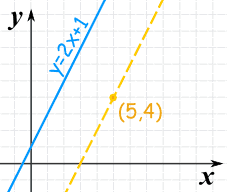+

# 1.5 - Linear Equations and Inequalities

##### Rating:
(2)
• (0)
• (2)
• (0)
• (0)
• (0)
Author: Kyle Webb
##### Description:

In Algebra, you learned about linear equations and inequalities.  Specifically, we can write the equation of a line if we have certain information:  two points, a point and the slope, or the y-intercept and the slope.  We have also learned three forms to use to write the equation of a line and when to use each form.  We will review these forms of equations of lines as well as look at linear inequalities in this section.

(more)
Tutorial

## Parallel and Perpendicular Lines

How do you know if two lines are parallel?

Their slopes are the same!How do you know if two lines are perpendicular?

When you multiply their slopes, you get -1

or The slopes are multiplicative inverses

or The perpendicular slope is the negative reciprocalRating Header